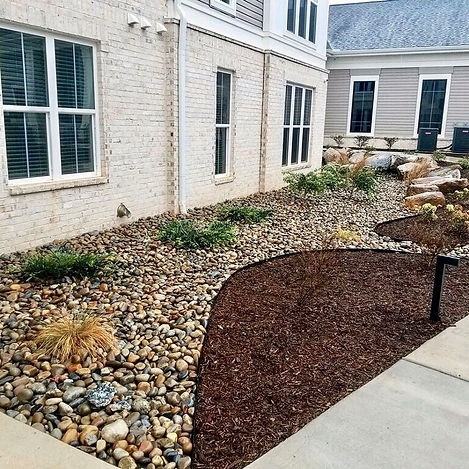top of page

If you have a rectangular shaped space utilize this calculator to better understand how much stone you will need for your project. You can also calculate rectangular boarders to outline your space.# Rectangular SpacesCircular spaces can be intimidating to calculate but it is very easy with our calculator tool. In this photo you can see a simple example of a circular space with a boarder.

# Circular Spaces

Some spaces are more unique and have different edges than a more tradition circle or rectangle. Utilize these calculators to measure out your unique space.# Unique Spaces# How Much Stone Do I Need?

Calculating how much stone or gravel you need for a project is very simple! You just need to calculate the dimensions of your space and input them into our calculator! You can calculate how many yards or tons you need, for any shape space!